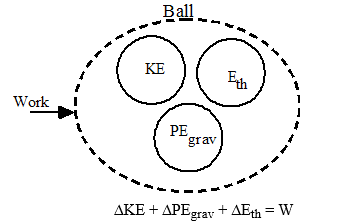# 2.7: Examples of Models

•• Dina Zhabinskaya
• UC Davis
$$\newcommand{\vecs}{\overset { \rightharpoonup} {\mathbf{#1}} }$$ $$\newcommand{\vecd}{\overset{-\!-\!\rightharpoonup}{\vphantom{a}\smash {#1}}}$$$$\newcommand{\id}{\mathrm{id}}$$ $$\newcommand{\Span}{\mathrm{span}}$$ $$\newcommand{\kernel}{\mathrm{null}\,}$$ $$\newcommand{\range}{\mathrm{range}\,}$$ $$\newcommand{\RealPart}{\mathrm{Re}}$$ $$\newcommand{\ImaginaryPart}{\mathrm{Im}}$$ $$\newcommand{\Argument}{\mathrm{Arg}}$$ $$\newcommand{\norm}{\| #1 \|}$$ $$\newcommand{\inner}{\langle #1, #2 \rangle}$$ $$\newcommand{\Span}{\mathrm{span}}$$ $$\newcommand{\id}{\mathrm{id}}$$ $$\newcommand{\Span}{\mathrm{span}}$$ $$\newcommand{\kernel}{\mathrm{null}\,}$$ $$\newcommand{\range}{\mathrm{range}\,}$$ $$\newcommand{\RealPart}{\mathrm{Re}}$$ $$\newcommand{\ImaginaryPart}{\mathrm{Im}}$$ $$\newcommand{\Argument}{\mathrm{Arg}}$$ $$\newcommand{\norm}{\| #1 \|}$$ $$\newcommand{\inner}{\langle #1, #2 \rangle}$$ $$\newcommand{\Span}{\mathrm{span}}$$$$\newcommand{\AA}{\unicode[.8,0]{x212B}}$$

## Video: Energy-Interaction Model with Mechanical Energies

### Example 1

Suppose we consider a baseball thrown by a pitcher. The pitcher’s hand exerts a force on the baseball over some distance parallel to the baseball’s velocity. Thus, the pitcher does a certain amount of work on the ball. Without knowing more details about how the ball was thrown, all we can say is that the work done by the pitcher's arm on the baseball equals the total change in energies of all other energy-systems of the ball. The natural way to model this situation is to treat the baseball as an open physical system.and W = F|| Dx, where ∆x is the distance the pitcher’s hand moved as s/he threw the ball parallel to the direction the ball traveled. This is about all we can say without having more information.

Perhaps we want to estimate the average force the pitcher exerts on the ball during the throw. If the throw is horizontal, there will be no change in gravitational potential energy. If we assume for now that we are focusing on the speed of the ball just after it is thrown, then air resistance will not cause significant energy to be transferred to thermal energy-systems. Thus, we can simplify the energy-system diagram to:In this simplified model, there is a more direct connection between work and kinetic energy. Since there is only one source of energy -- the work the pitcher does -- and there is only one form the energy ends up taking -- kinetic energy, the two can be equated. Writing it out:

$$\Delta KE = \dfrac{1}{2}mv_{f}^{2}$$ $$W = F_{avg} \cdot \Delta x$$ AND $$\Delta KE = W$$

Therefore:

$F_{avg} \cdot \Delta x = \dfrac{1}{2}mv_{f}^{2}$

So, if we knew how fast the ball was thrown (which gives us ∆KE), we can determine a value for the force exerted on the ball by the pitcher by making a reasonable estimate for the distance ∆x. If we do this and solve for $$F_{avg}$$, we would find that

$F_{avg} = \dfrac{mv_{f}^{2}}{2 \Delta x}$

Exercise 1

Using your own estimates for physical values, what average force does a pitcher exert when throwing a fastball? (~90mph, or 40.2m/s)

How much acceleration does the ball experience?

Challenge: Using this acceleration, about how long did it take for the pitcher to complete the throw? (Hint: $$a= \dfrac{\Delta v}{\Delta t}$$)

### Example 2

Instead of being interested in the force the pitcher exerts on the ball when s/he throws it, we might be interested in how much energy the pitcher uses to make a large number of throws. In this case the natural approach is to include the pitcher in the energy-system diagram and not focus on the work done by the pitcher.

We create a closed physical system consisting of the pitcher and the ball.

before: ball at rest after: ball with velocity vThe change in energy within the system is always zero, so however much energy is gained by the balls must have been lost by the pitcher.

$\Delta E_{pitcher} + \Delta KE_{ball} = 0$

Therefore

$\Delta E_{pitcher} = - \dfrac{1}{2}mv_{f}^{2}$

for each pitch.

Note the sign of the change in energy of the pitcher. Does this make sense? You should be able to calculate how many food (big C) calories the pitcher burns after throwing say 100 pitches with the ball averaging 50 miles per hour as it leaves the pitcher’s hand.

Exercise 2

Is this a good way to stay slim and still eat large hamburgers with fries?

# Contributors

This page titled 2.7: Examples of Models is shared under a not declared license and was authored, remixed, and/or curated by Dina Zhabinskaya.